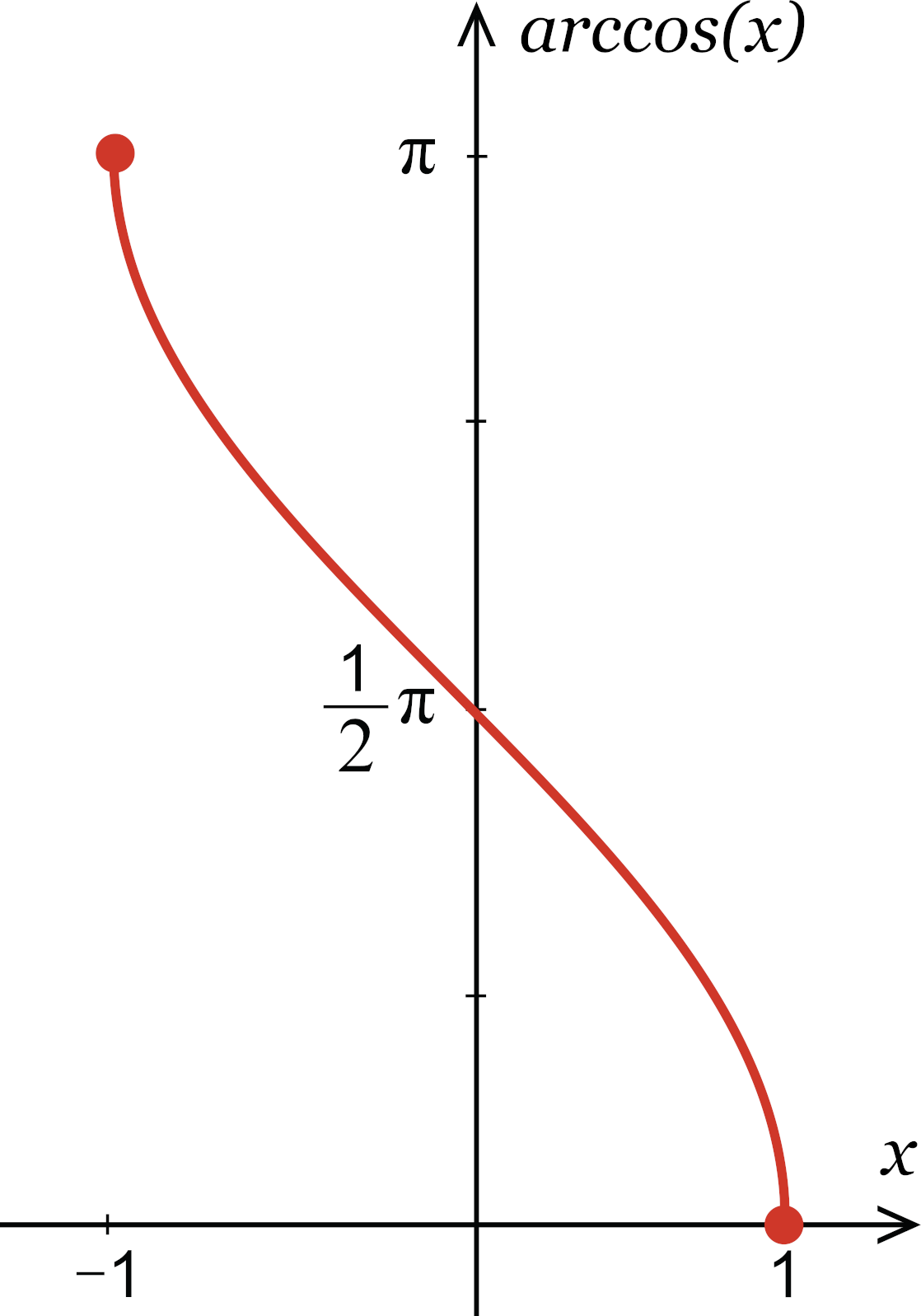# Inverse Cosine Calculator – Calculate arccos(x)

Find the angle in degrees or radians using the inverse cosine with the arccos calculator below.

## How to Find Arccos

Arccos is a trigonometric function to calculate the inverse cosine. Arccos can also be expressed as cos-1(x).

The term inverse means the opposite or to “undo” something. For example, addition and subtraction or inverse operations.

Arccos is used to undo or reverse the cosine function. If you know the cosine of an angle, you can use arccos to calculate the measurement of an angle.

Since arccos is the inverse of the cosine function, and many angles share the same cosine value, arccos is a periodic function. Each arccos value can result in multiple angle values, which is why the domain is restricted to [-1, 1]. The primary result for arccos is known as the principal value and is the angle in the range of 0° to 180°.

To calculate arccos, use a scientific calculator and the acos or cos-1 function, or just use the calculator above. Most scientific calculators require the angle value in radians to solve for cos.

### Inverse Cosine Formula

The inverse cosine formula is:

y = cos(x) | x = arccos(y)

Thus, if y is equal to the cosine of x, then x is equal to the arccos of y.

## Inverse Cosine Graph

If you graph the arccos function for every possible value of cosine, it forms a decreasing curve from (-1, π) to (1, 0).Because the value of the cosine function oscillates in the range of -1 to 1, the inverse cosine curve’s domain starts at x = -1 and ends at x = 1. Since the peak (maximum) of the cosine wave is at 0 radians and the dip (minimum) of the wave is at π radians, the y value ends at those points.

## Inverse Cosine Table

The table below shows common cosine values and the arccos, or angle for each of them.

Table showing common cosine values and inverse cosine values for each in degrees and radians
-1 180° π
√6 + √2 / 4 165° 11π / 12
√3 / 2 150° / 6
√2 / 2 135° / 4
1 / 2 120° / 3
√6 – √2 / 4 105° / 12
0 90° π / 2
√6 – √2 / 4 75° / 12
1 / 2 60° π / 3
√2 / 2 45° π / 4
√3 / 2 30° π / 6
√6 + √2 / 4 15° π / 12
1 0

You might also be interested in our inverse sine and inverse tangent calculators.

## How to Use Inverse Cosine to Find an Angle in a Right Triangle

You can find the angle in a right triangle by finding the arccosine.

Begin by identifying and labeling the hypotenuse, opposite side, and adjacent side in regards to the angle you want to find.

Use the equation y = arccos(adjacent/hypotenuse) and evaluate.

If the opposite side and hypotenuse are known, you can find the value of y directly and round the answer to the nearest degree or decimal place.

If the opposite side and hypotenuse are not known, you can use the Pythagorean theorem to find the missing side lengths before using the above formula.

### What is the relationship between arccos and arcsin?

Arcsine and arccosine are complementary to one another due to the fact that the arcsine function gives us the angle whose sine is a given ratio. This can be seen with the expression: ​

arcsin(x) = π/2 – arccos(x)

The arcsin function gives us the angle whose sine is a given ratio, while the arccos function gives us the angle whose cosine is a given ratio. These angles are different in a right triangle, but they are always complementary, meaning that their sum is always 90 degrees (or π/2 radians).

### What is cosine to the power of -1?

Cosine-1, also written as arccosine or acos, refers to the inverse cosine function. This function takes a value between -1 and 1 as the input and returns an angle in radians as the output.

For example, if cosine(x) = 0.5, then cosine-1(0.5) = 1.047 radians. This is approximately 60 degrees, which means that the angle whose cosine is 0.5 is 60 degrees or 1.047 radians.

### Can you find the inverse of cosine without a calculator?

Yes, you can find the inverse cosine, or arccosine, without a calculator by identifying the value that you want to find the inverse cosine for. Then write down the equation cos(y) = x and solve for y by taking the arcosine of both sides of the equation.

You can then evaluate the expression using algebraic methods for simple fractions or geometric methods for more complex values. Some values, however, may require you to use the table of trigonometric values.

### What is the inverse cosine of 0?

The inverse cosine of 0 is π/2 radians or 90 degrees. This is because the cosine function has a maximum value of 1 at 0 radians and the inverse cosine function takes a value of 0 at π/2 radians, which is the midpoint of the cosine function’s range. Thus, cos-1(0) = π/2 radians or 90 degrees.

### Is the inverse cosine the same as 1 over cos?

Although this is a common mistake, inverse cosine is not the same as 1/cosine. Arccosine is the inverse of the cosine function where 1/cosine is the reciprocal of the cosine.